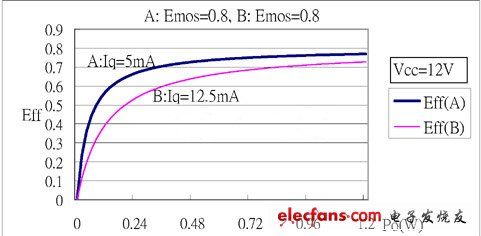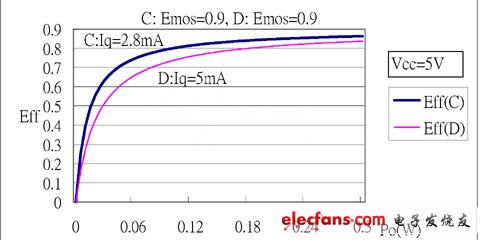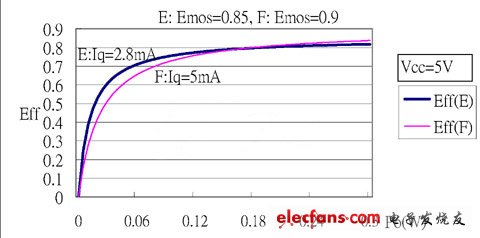# Class D audio amplifier efficiency and interference in use

From the previous discussion of "how to evaluate the dynamic efficiency of Class D audio amplifiers", if Poq represents the ratio of output power Po to static power consumption Pq, Emos represents the efficiency of the output power transistor and Eff represents the total efficiency, then the total efficiency:

Eff = (Poq x Emos) / (Poq + Emos)

The above formula shows that the influence of Poq and the total efficiency is the same as that of the output power transistor efficiency Emos and the total efficiency, so the static power consumption Pq or the static current consumption Iq needs to be considered when evaluating the total efficiency.Figure 1

Figure 1 shows the Eff curves of two stereo class-D amplifiers. The power supply of these two amplifiers is 12V and the efficiency of the output transistor Emos is 0.8. The quiescent current of curve A amplifier is 10mA and the quiescent current of curve B amplifier is 25mA. Because it is stereo, the quiescent current of each single channel is only half, that is, the quiescent current of curve A is 5mA and the curve B is 12.5mA. It can be seen from the graph that the total efficiency of curve A is significantly better than curve B when the output power is low or the output power is about 10% or less of the maximum output power. Curve A is the Eff curve of TMPA430DS at 12V and 8ohm resistive load.Figure II

Figure 2 shows the Eff curves of two stereo Class D amplifiers. The power supply of these two amplifiers is 5V and the efficiency of the output transistor Emos is 0.9. The quiescent current of curve C amplifier is 5.6mA and the quiescent current of curve B amplifier is 10mA. So the quiescent current of each channel of curve C is 2.8mA and curve D is 5mA. It can be seen from the graph that the pros and cons of the two curves are equivalent to those in Figure 1. A low quiescent current has a high total efficiency Eff. Curve C is the Eff curve of TMPA3155DS at 5V and 8ohm resistive load.Figure three

Figure 3 also shows the Eff curves of two stereo class-D power amplifiers. Curve F and curve D of Figure 2 are the same power amplifier. Curve E and curve C of Figure 2 have the same conditions except that the transistor efficiency is only 0.85. Figure 3 shows that although the efficiency of the transistor E is only 0.85 lower than 0.9 of the curve F, but because the quiescent current of the curve E is 2.8mA is lower than the 5mA of the curve F, the total efficiency of the curve E is higher than the output power less than 0.18W , Which emphasizes the effect of low quiescent current on the total efficiency Eff.

Product application:
â– Products for commercial,household,lighting

Product features:
â– High strength,impact resistance and thermal stability
--Panel material using high quality polycarbonate
â– High resistance to deformation
---Long term use without obvious distortion
----The flame retardant grade of panel is in line with UL94-V2